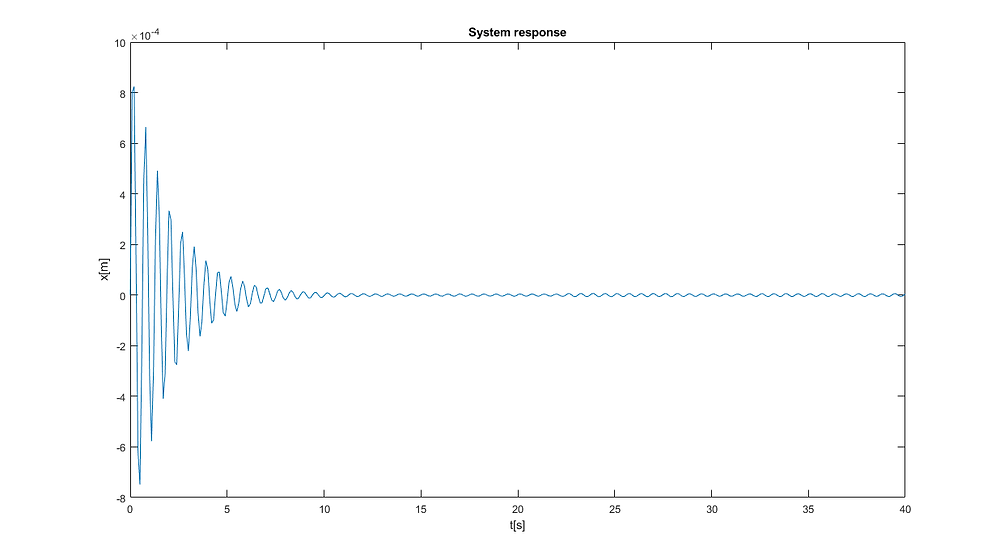Search

# Solving an Ordinary Differential Equation in MATLAB with the ode45-solver

Updated: Jan 5, 2020

In this short post I will show you how to solve a second order ordinary differential equation with MATLAB.System response of a single mass oscillator

The goal is to separate a given differential equation of n-th order in n differential equations of first order. The following ODE for a single mass oscillator with damping d, external excitation F und spring stiffness c is given:Differential equation for a single mass oscillator

A complete instruction can be found here: https://drive.google.com/file/d/1v9VNQsWhrDMLiVfgQL6aLLmJ2zguhdMk/view?usp=sharingIn case you have any questions, feel free to reach out to me! The code for multiple plots and for solving the ODE can be found in my Github repo.

Jousef